# Test: Semiconductor Electronics 2 - From Past 28 Years Questions

## 25 Questions MCQ Test Physics 28 Years Past year papers for NEET/AIPMT Class 12 | Test: Semiconductor Electronics 2 - From Past 28 Years Questions

Description
This mock test of Test: Semiconductor Electronics 2 - From Past 28 Years Questions for Class 12 helps you for every Class 12 entrance exam. This contains 25 Multiple Choice Questions for Class 12 Test: Semiconductor Electronics 2 - From Past 28 Years Questions (mcq) to study with solutions a complete question bank. The solved questions answers in this Test: Semiconductor Electronics 2 - From Past 28 Years Questions quiz give you a good mix of easy questions and tough questions. Class 12 students definitely take this Test: Semiconductor Electronics 2 - From Past 28 Years Questions exercise for a better result in the exam. You can find other Test: Semiconductor Electronics 2 - From Past 28 Years Questions extra questions, long questions & short questions for Class 12 on EduRev as well by searching above.
QUESTION: 1

### Reverse bias applied to a junction diode 

Solution:

In reverse biasing, the conduction across the
p-n junction does not take place due to
majority carriers but takes place due to
minority carriers if the voltage of external
battery is large. The size of the depletion
region increases thereby increasing the
potential barrier.

QUESTION: 2

### In semiconductors, at room temperature 

Solution:

In a semiconductor, at room temperature, few of the electrons in the valence band gain enough energy to jump into the conduction band whereas the others remain in the valence band. Hence, the valence band is partially empty and the conduction band is partially filled.

QUESTION: 3

### The peak voltage in the output of a half-wavediode rectifier fed with a sinusoidal signalwithout filter is 10V. The d.c. component of theoutput voltage is 

Solution: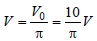QUESTION: 4

The output of OR gate is 1  

Solution:

Output will be one if A or B or both are one.
Y= A + B

QUESTION: 5

In a p-n junction photocell, the value of thephoto-electromotive force produced bymonochromatic light is proportional to 

Solution:

Electromotive force depends upon intensity
of light falling, it does not depend on
frequency of barrier voltage

QUESTION: 6

Of the diodes shown in following diagrams, which one is reverse biased ? 

Solution:

Positive terminal is at lower potential (0V)
and negative terminal is at higher potential
+5 V

QUESTION: 7

Choose the only false statement from thefollowing 

Solution:

(a) is true as in case of conductors either the
conduction & valence band overlap or
conduction band is partially filled.
(b) is true as insulators have energy gap of
the order of 5 to 10 eV.
(c) is false as resistivity (opposite of
conductivity) decreases with increase in
temperature.
(d) is true as with increase in temperature
conduction band. So, conductivity increases.

QUESTION: 8

Carbon, silicon and germanium atoms have fourvalence electrons each. Their valence andconduction bands are separated by energy bandgaps represented by (Eg)C, (Eg)Si and (Eg)Gerespectively. Which one of the followingrelationships is true in their case? 

Solution:

Due to strong electronegativity of carbon

QUESTION: 9

Application of a forward bias to a p–n junction 

Solution: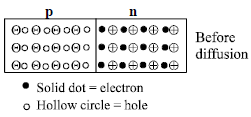In forward bias, p-type is connected to positive terminal of battery and n-type is connected to negative terminal of battery. Hence, carriers are accelerated towards opposite terminals for the conduction thereby increases the number of electrons on n-side.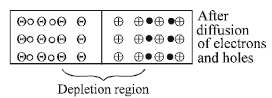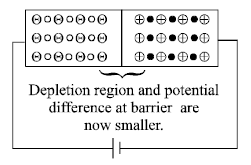Number of donor s is more because
electrons from –ve terminal of the cell
pushes (enters) the n side and decreases
the number of uncompensated pentavalent
ion due to which potential barrier is
reduced. The neutralised pentavalent atom
are again in position to donate electrons.

QUESTION: 10

Zener diode is used for 

Solution:

At a certain reverse bias voltage, zener diode
allows current to flow through it and hence
maintains the voltage supplied to any load
Hence, it is used for stabilisation.

QUESTION: 11

The following figure shows a logic gate circuit with two inputs A and B and the output C. The voltage waveforms of A, B and C are as shown below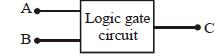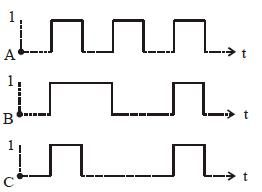The logic circuit gate is 

Solution:

On the basis of given graph, following table
is possible.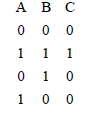It is the truth table of AND gate.

QUESTION: 12

A transistor is operated in common emitterconfiguration at constant collector voltage Vc =1.5V such that a change in the base current from100 μA to 150 μA produces a change in thecollector current from 5 mA to 10 mA. The currentgain (β) is 

Solution: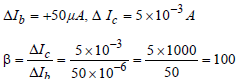QUESTION: 13

A forward biased diode is     

Solution:

In forward biasing of a diode, the emitted
should be at a higher potential, Here, only in
option (c) emitter is at higher potential with
bias voltage 0V compared to the collector,
which is at –2V. So, it is reverse biased.

QUESTION: 14

The radius of germanium (Ge) nuclide ismeasured to be twice the radius of 94Be . Thenumber of nucleons in Ge are 

Solution:

We use the formula,
R = R0 A1/3
This represents relation between atomic mass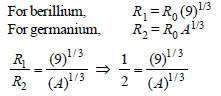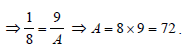QUESTION: 15

In the following circuit, the output Y for all possible inputs A and B is expressed by the truth table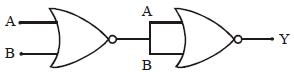Solution: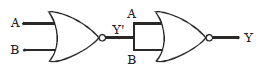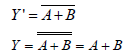Therefore truth table :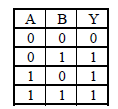QUESTION: 16

A common emitter amplifier has a voltage gainof 50, an input impedance of 100Ω and an outputimpedance of 200Ω. The power gain of theamplifier is 

Solution: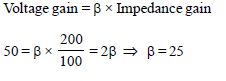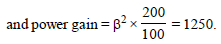QUESTION: 17

For a cubic crystal structure which one of thefollowing relations indicating the cellcharacteristics is correct? 

Solution:

For a cubic crystal,
a = b = c and α = β = γ = 90°

QUESTION: 18

In the energy band diagram of a material shown below, the open circles and filled circles denote holes and electrons respectively. The material is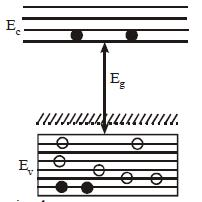Solution:

For a p-type semiconductor, the acceptor
energy level, as shown in the diagram, is
slightly above the top Ev of the valence band.
With very small supply of energy an electron
EA and ionise acceptor negatively.

QUESTION: 19

The voltage gain of an amplifier with 9% negativefeedback is 10. The voltage gain withoutfeedback will be 

Solution:

Negative feedback is applied to reduce the
output voltage of an amplifier. If there is no
negative feedback, the value of output
voltage could be very high. In the options
given, the maximum value of voltage gain is
100. Hence it is the correct option.

QUESTION: 20

A p-n photodiode is made of a material with aband gap of 2.0 eV. The minimum frequency ofthe radiation that can be absorbed by the materialis nearly 

Solution:

Eg = 2.0 eV = 2 × 1.6 × 10–19 J
Eg = hv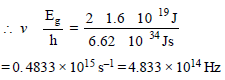QUESTION: 21

The circuitis equivalent to

Solution:

Let A and B be inputs and Y the output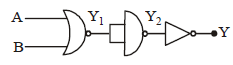Then Y1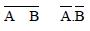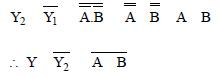Hence, the given circuit is equivalent to a
NOR gate.

QUESTION: 22

The symbolic representation of four logic gates are given below :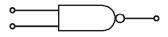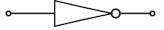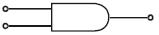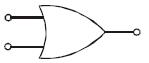The logic symbols for OR, NOT and NAND gates are respectively:

Solution:

(iv), (ii), (i)

Option (iii) represents AND gate.

QUESTION: 23

A p-n photodiode is fabricated from a semiconductor with a band gap of 2.5 eV. It candetect a signal of wavelength 

Solution: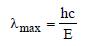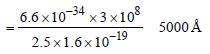The wavelength detected by photodiode
should be less than λmax. Hence it can
detect a signal of wavelength 4000Å.

QUESTION: 24

A transistor is operated in common-emitterconfiguration at Vc = 2 V such that a change inthe base current from 100 μA to 200 μA producesa change in the collector current from 5 mA to10 mA. The current gain is 

Solution: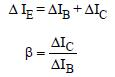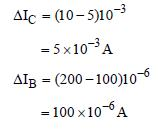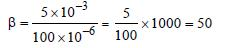QUESTION: 25

The number of beta particles emitted by aradioactive substance is twice the number ofalpha particles emitted by it. The resultingdaughter is an 

Solution:

Isotopes of an element have the same atomic
number but different mass number. A
radioactive substance when emits one alpha
particles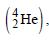its mass number reduces
by 4 and charge no. reduces by 2 and after
emission of two β-particles its charge no.
increase by 2 thus the charge no. i.e. atomic
number remains the same.